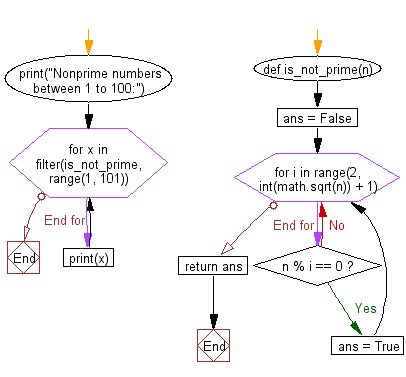﻿ Python: Identify nonprime numbers between 1 to 100 - w3resource# Python: Identify nonprime numbers between 1 to 100

## Python Basic - 1: Exercise-116 with Solution

Write a Python program to identify nonprime numbers between 1 to 100 (integers). Print the nonprime numbers.

Sample Solution:

Python Code:

``````import math
def is_not_prime(n):
ans = False
for i in range(2, int(math.sqrt(n)) + 1):
if n % i == 0:
ans = True
return ans
print("Nonprime numbers between 1 to 100:")
for x in filter(is_not_prime, range(1, 101)):
print(x)
``````

Sample Output:

```Nonprime numbers between 1 to 100:
4
6
8
9
10
12
14
15

..

90
91
92
93
94
95
96
98
99
100
```

Flowchart:Python Code Editor:

Have another way to solve this solution? Contribute your code (and comments) through Disqus.

What is the difficulty level of this exercise?

Test your Programming skills with w3resource's quiz.

﻿

## Python: Tips of the Day

What is the difference between Python's list methods append and extend?

append: Appends object at the end.

```x = [1, 2, 3]
x.append([4, 5])
print (x)
```

Output:

```[1, 2, 3, [4, 5]]
```

extend: Extends list by appending elements from the iterable.

```x = [1, 2, 3]
x.extend([4, 5])
print (x)
```

Output:

```[1, 2, 3, 4, 5]
```

Ref: https://bit.ly/2AZ6ZFq